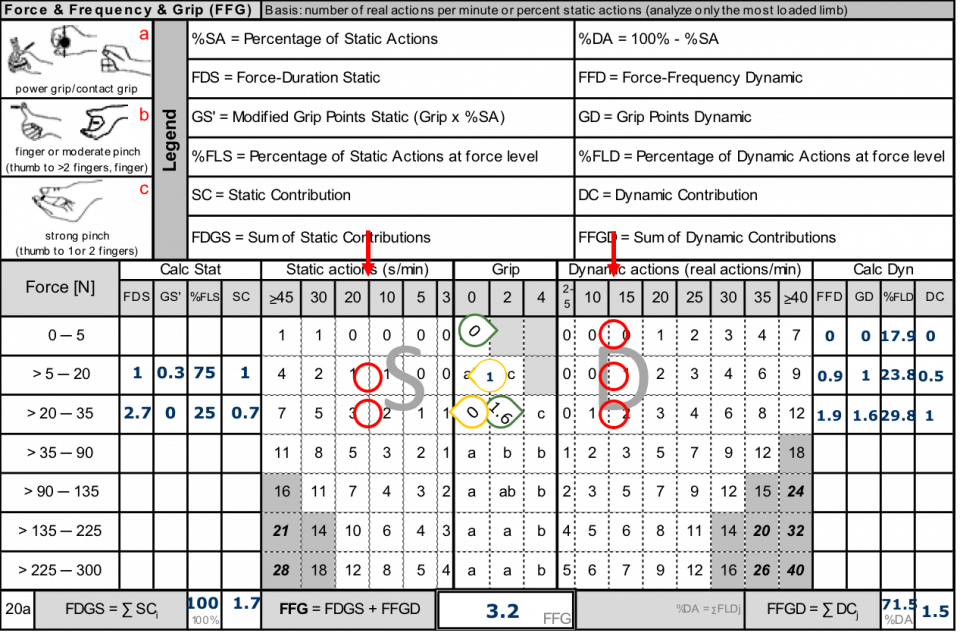# Solution

Number of dynamic actions = 3 + 4 + 2 + 3 = 12 RA

Duration of static actions = 5 + 15 =20 s

Cycle time ≠ 60 s --> conversion necessary

•   %SA per minute = 20 s/70 s × 100 % = 28.57 % 28.6 %
• 20 seconds of static action DSA = 20/70 × 60 = 17.1 s/min

•  %DA per minute =100 - 28.6 = 71.4%
• 12 dynamics actions FDA = 12/(70-20) × 60 = 14.4 actions/min

Dynamic actions:

Force level 1

• Scoring at level 1 from table at 14.4 actions = 0 Points
• Grip scoring c at level 1 GD1 = 0 Points
• Level 1 percentage FLD1 = 3/12 × 50/70 = 17.9 %

Force level 2

• Scoring at level 2 from table at 14.4 actions = 0.9 Points
• Grip scoring b at level 2 GD2 = 1 Point
• Level 2 percentage FLD2 = 4/12 × 50/70 = 23.8%

Force level 3

• Scoring at level 3 from table at 14,4 actions = 1.9 Points
• Grip scoring at level 3 - GD3: (2×4+3×0)/5 = 1.6 Points
• Level 3 percentage FLD3 = 5/12 × 50/70 = 29.8 %

Static actions:

20 s = 17.1 s/min; %SA = 20 s/70 s × 100% = 28.6 %

Force level 2:

• FDS2: 1 point
• grip point b GS2 = 1 GS’2= 1 × 28.6 % = 0.3 Points
• %FLS2 : 15/20 = 75%

Force level 3:

• FDS3: 2.7 points
• grip points a GS3 = 0 GS’3 = 0 × 28,6% = 0 Points
• %FLS3 : 5/20 = 25%

SC2 = 75% × (1 + 0.286) = 1.0 Point

SC3 = 25% × (2.7 + 0)= 0.7 Points